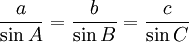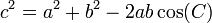# Solving Triangles

"Solving" means finding missing sides and angles.When we know any 3 of the sides or angles ... ... we can find the other 3 (Except for only 3 angles, because we need at least one side to find how big the triangle is.)

## Six Different Types

If you need to solve a triangle right now choose one of the six options below:

Which Sides or Angles do you know already? (Click on the image or link)AAS

Two Angles and a Side not betweenASA

Two Angles and a Side betweenSAS

Two Sides and an Angle betweenSSA

Two Sides and an Angle not between

... or read on to find out how you can become an expert triangle solver:

## Your Solving Toolbox

Want to learn to solve triangles?

Imagine you are "The Solver" ...
... the one they ask for when a triangle needs solving!

In your solving toolbox (along with your pen, paper and calculator) you have these 3 equations:

### 1. The angles always add to 180°:

A + B + C = 180°

When you know two angles you can find the third.

### 2. Law of Sines (the Sine Rule):When there is an angle opposite a side, this equation comes to the rescue.

Note: angle A is opposite side a, B is opposite b, and C is opposite c.

### 3. Law of Cosines (the Cosine Rule):This is the hardest to use (and remember) but it is sometimes needed
to get you out of difficult situations.

It is an enhanced version of the Pythagoras Theorem that works
on any triangle.

With those three equations you can solve any triangle (if it can be solved at all).

## Six Different Types (More Detail)

There are SIX different types of puzzles you may need to solve. Get familiar with them:

### 1. AAA:

This means we are given all three angles of a triangle, but no sides.AAA triangles are impossible to solve further since there are is nothing to show us size ... we know the shape but not how big it is.

We need to know at least one side to go further. See Solving "AAA" Triangles .

### 2. AAS

This mean we are given two angles of a triangle and one side, which is not the side adjacent to the two given angles.Such a triangle can be solved by using Angles of a Triangle to find the other angle, and The Law of Sines to find each of the other two sides. See Solving "AAS" Triangles.

### 3. ASA

This means we are given two angles of a triangle and one side, which is the side adjacent to the two given angles.In this case we find the third angle by using Angles of a Triangle, then use The Law of Sines to find each of the other two sides. See Solving "ASA" Triangles .

### 4. SAS

This means we are given two sides and the included angle.For this type of triangle, we must use The Law of Cosines first to calculate the third side of the triangle; then we can use The Law of Sines to find one of the other two angles, and finally use Angles of a Triangle to find the last angle. See Solving "SAS" Triangles .

### 5. SSA

This means we are given two sides and one angle that is not the included angle.In this case, use The Law of Sines first to find either one of the other two angles, then use Angles of a Triangle to find the third angle, then The Law of Sines again to find the final side. See Solving "SSA" Triangles .

### 6. SSS

This means we are given all three sides of a triangle, but no angles.In this case, we have no choice. We must use The Law of Cosines first to find any one of the three angles, then we can use The Law of Sines (or use The Law of Cosines again) to find a second angle, and finally Angles of a Triangle to find the third angle. See Solving "SSS" Triangles .

## Tips to Solving

Here is some simple advice:

When the triangle has a right angle, then use it, that is usually much simpler.

When two angles are known, work out the third using Angles of a Triangle Add to 180°.

Try The Law of Sines before the The Law of Cosines as it is easier to use.